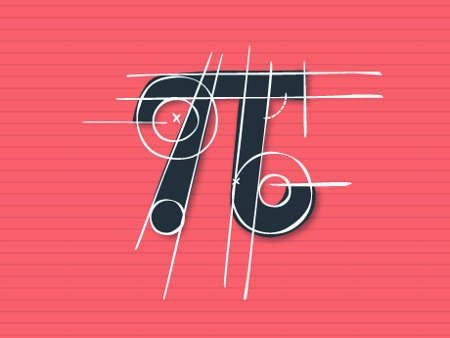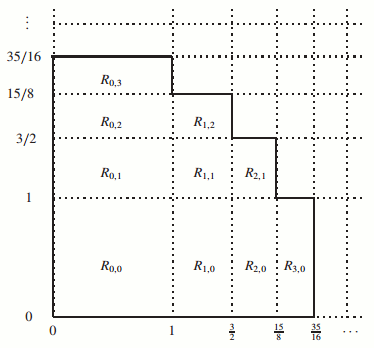### Pi day: Zilienski, Wallis and the square

http://t.co/SopVLuLJHM by @ulaulaman #piday
A good pi day to all readers! I hope that the following post, that I cannot review after the first writing, could be interesting to all!The technique used by the ancient Greek for their geometric constructions was called "ruler and compass". In this way you can build a lot of regular polygons, for example, but there are three problems that are impossible unless you use different techniques: the angle trisection, doubling the cube, squaring the circle.
In particular for the squaring, it is easy to calculate the relation between the radius $r$ of the circke and the side $l$ of the square with the same area of the starting circle: $L = \sqrt {\pi} r$ Now, since $\pi$ is a transcendental number, the formula above is the simplest representation of the impossibility of squaring the circle using ruler and compass: with these devices you can treat rational and irrational numbers, such as $\sqrt{2}$ (in this case simply draw a square of side 1).
So, using these two tools it is possible to obtain an approximate construction and, therefore, a corresponding approximate value for $\pi$: during the XX century there are produced a lot of approximations, for example by CD Olds (1963), Martin Gardner (1966), Benjamin Bold (1982). They are all variations of the geometric construction discovered by Srinivasa Ramanujan in 1913, that approached $\pi$ with the fraction $\frac{355}{113} = 3.1415929203539823008 \dots$ right up to the sixth decimal place.
In 1914, Ramanujan discovered a more accurate approximation (to eight decimal places), always using ruler and compass: $\left (9^2 + \frac{19}{22}^2 \right)^{1/4} = \sqrt{\frac{2143}{22}} = 3.1415926525826461252 \dots$ If however, to compass and ruler we add a bit of mechanics, we can get an interesting way to square the circle (with a subsequent measure of $\pi$!). Take a circle of radius $r$ and let's roll with constant speed along its tangent line. The point of tangency $N$ describe a curve called a cycloid.
Now you can construct a second cycloid using the projection of the point $M$ on the tangent line. The intersection $B$ between the diameter $NN'$ with this second cycloid will provide a segment $NB$ whose length is equal to $\sqrt{\pi} r$, which is the side of the square with the same area of the starting circle. In this way, using a bit of algebra, is it also possible to determine the value of $\pi$.
But this construction is not simply geometric, but we can also do it really, perhaps with a small brass disc as suggested by August Zielinski, the proponent of the method of squaring just now briefly described.
Obviously in this case the precision of the value of $\pi$ depends on the accuracy of the devices used.
Papers by Ramanujan:
Squaring the circle, Journal of the Indian Mathematical Society, V, 1913, 132
Modular equations and approximations to $\pi$, Quarterly Journal of Mathematics, XLV, 1914, 350 – 372
Zielinski A. (1875). Quadrature of the Circle, The Analyst, 2 (3) 77. DOI: http://dx.doi.org/10.2307/2635871 (archive.org)
Crop Circles and More | McTutor | Squaring the circle: a history of the problem by E. W. Hobson
Among the many formulas used to calculate $\pi$, a special place is held by the Wallis product, discovered more or less by an accident by the mathematician John Wallis, as he was trying to calculate the area of ​​a circle. $\prod_{n=1}^\infty \left ( \frac{2n}{2n-1} \cdot \frac{2n}{2n+1} \right ) = \frac{2}{1} \cdot \frac{2}{3} \cdot {4}{3} \cdot \frac{4}{5} \cdots = \frac{\pi}{2}$ Wallis presented his formula in his most famous book, Arithmetica infinitorum (arithmetic of the infinite) of 1665, essential for the Isaac Newtons's training, who became his successor as a landmark of British mathematicians.
However, the history of the formula began in Italy in 1632 with the publication of Lo Specchio Ustorio, overo, Trattato delle settioni coniche (the burning glass, or a treatise about conic sections) where the mathematician Bonaventura Cavalieri calculates the area under "parables" of type $y = x^n$. Cavalieri proceeded by calculating the area between the axes and curves of type $y = \left ( 1-x^2 \right )^0, \; y= \left ( 1-x^2 \right )^1 \; y= \left ( 1-x^2 \right )^2\; y= \left ( 1-x^2 \right )^3, \; \cdots$ obtaining $x,$ $x - \frac{1}{3} x^3,$ $x - \frac{2}{3} x^3 + \frac{1}{5} x^5,$ $x - \frac{3}{3} x^3 + \frac{3}{5} x^5 - \frac{1}{7} x^7,$ $x - \frac{4}{3} x^3 + \frac{6}{5} x^5 - \frac{4}{7} x^7 + \frac{1}{9} x^9,$ $\cdots$ Since the equation of the circle of radius 1 is $y = \left (1-x^2 \right)^{1/2}$, the problem reduces to determining the expression corresponding to the exponent $\frac{1}{2}$ between $x$ and $x - \frac{1}{3} x^3$.
Unable to determine the expression, Wallis completed a series of numerical calculations that led him eventually to the formula that bears his name.A more or less simple proof of the formula passes through the definition of three new series and the numerical calculation of the area of ​​rectangles in the Cartesian plane: already just looking at the picture below is easy to understand how these series, approximating the rectangles, to the limit they approach the arc of a circle, so converging to the value of $\frac{\pi}{2}$.
A more rigorous demonstration, however, that takes the road of the proof of the formula of Cavalieri, passes through the integral of the breast $\int_0^\frac{\pi}{2} \sin^n x dx$ It is also possible, using suitably the Dirichlet eta function, to determine a link between the Riemann zeta and the formula of Wallis, which is also quite obvious because the Riemann zeta is linked to pi.
Wildberger N.J. (2002). A New Proof of Cavalieri's Quadrature Formula, The American Mathematical Monthly, 109 (9) 843. DOI: http://dx.doi.org/10.2307/3072373
Young R.M. (1998). Probability, pi, and the Primes: Serendipity and Experimentation in Elementary Calculus, The Mathematical Gazette, 82 (495) 443. DOI: http://dx.doi.org/10.2307/3619891
Wästlund J. (2007). An Elementary Proof of the Wallis Product Formula for pi, The American Mathematical Monthly, 114 (10) 914-917. DOI: http://dx.doi.org/10.2307/27642364 (pdf)
Reading also: Mathworld | The world of $\pi$ | Math fun facts